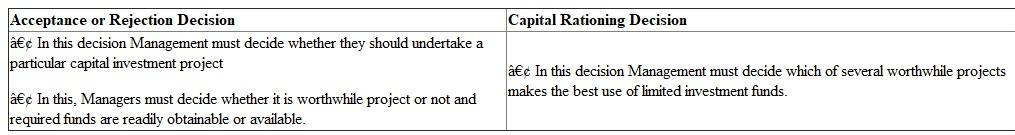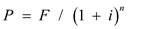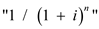# Quiz 16: Capital Expenditure Decisions

Distinction between the following two types of capital-budgeting decisions: Acceptance or Rejection Decisions and capital-Rationing DecisionLower the Discount, Greater is the Present Value of Future Cash Flow: The value of future money can be calculated to present worth or present value with the "discount rate" asWhere: F = future cash flow (positive for receipts, negative for disbursements) PV = present value i = discount rate n = number of interest periods The factoris known as the "single payment present worth factor". Discount Rate The interest rate utilized in the discounted cash flow analysis for determining the existing value of the future cash flows. The discount rate takes into account time value of the money (the idea is that money accessible now is worth more than same amount of money obtainable in future as it might be earning interest) and risk or uncertainty for anticipated future cash flows (that might be less than anticipated). Apart from other uses, discount rates are used for calculating value of the future income streams to present day. The discount rate will differ in the lost profit analysis, where income stream can be less assured, depending on the degree of risk related with the income stream. For determining the correctness of statement as well as measure dependency of the discount rates as well as present value let's assume as per the above formulae the following discount rates: Future Income stream of \$100,000 and discount rates = 4 %, 8 % and 12 % respectively Discount Rate Present Value Estimate 4% ……………………………………… \$811,090 8% ……………………………………… \$671,008 12% …………………………………….. \$565,022 Therefore the lower the discount rate the higher is the present value of future cash flows.